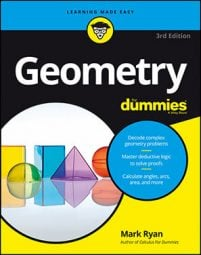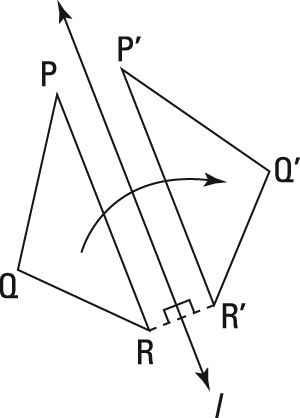##### Geometry For DummiesWhen you reflect a shape in coordinate geometry, the reflected shape remains congruent to the original, but something changes. That something is the new shape's orientation.

For example, as you can see in the image, the triangle in the mirror is flipped over compared with the real triangle.

Mirrors (and mathematically speaking, reflections) always produce this kind of flipping. Flipping a figure switches its orientation.

In the second figure, triangle PQR has been reflected across line l to produce triangle P'Q'R'.Reflecting triangle PQR over line l switches the figure's orientation.

Triangles PQR and P'Q'R' are congruent, but their orientations are different:

• One way to see that they have different orientations is that you can't get triangles PQR and P'Q'R' to stack on top of each other—no matter how you rotate or slide them—without flipping one of them over.
• A second characteristic of figures with different orientations is the clockwise/counterclockwise switch. Notice that in triangle PQR, you go counterclockwise from P to Q to R, but in the reflected triangle, triangle P'Q'R', you go clockwise from P' to Q' to R'.
The reflection shown in the second figure can be thought of as a folding. If you were to fold this image along line l, triangle PQR would end up stacked perfectly on triangle P'Q'R', with P on P', Q on Q', and R on R'.

Reflections and orientation. Reflecting a figure once switches its orientation. When you reflect a figure more than once, the following rules apply:

• If you reflect a figure and then reflect it again over the same line or a different line, the figure returns to its original orientation. More generally, if you reflect a figure an even number of times, the final result is a figure with the same orientation as the original figure.
• Reflecting a figure an odd number of times produces a figure with the opposite orientation.